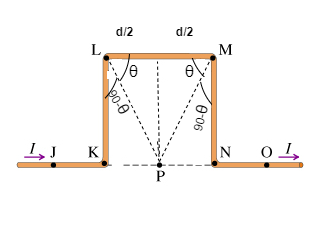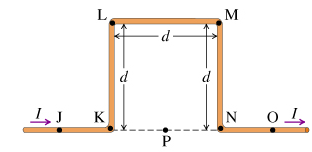# Problem: A long wire carries current I. The wire is straight except for one square-shaped "half-loop" KLMN as shown (Figure 1). Assume that d is a known quantity.(a) Find the magnitude of magnetic field Bnet at point P. Express your answer in terms of some or all of the quantities d and /, and the constants  μ0 and π.(b) Find the direction of the magnetic field at P.

###### FREE Expert Solution

Magnetic field:

$\overline{){\mathbf{B}}{\mathbf{=}}\frac{{\mathbf{\mu }}_{\mathbf{0}}\mathbf{I}}{\mathbf{2}\mathbf{\pi }\mathbf{r}}}$

Consider(a) BJK = 0 T

80% (1 ratings)###### Problem Details

A long wire carries current I. The wire is straight except for one square-shaped "half-loop" KLMN as shown (Figure 1). Assume that d is a known quantity.(a) Find the magnitude of magnetic field Bnet at point P. Express your answer in terms of some or all of the quantities d and /, and the constants  μ0 and π.
(b) Find the direction of the magnetic field at P.